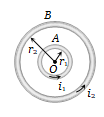A and B are two concentric circular conductors of centre O and carrying currents and ${\mathrm{i}}_{2}$ as shown in the adjacent figure. If ratio of their radii is 1 : 2 and ratio of the flux densities at O due to A and B is 1 : 3, then the value of ${\mathrm{i}}_{1}/{\mathrm{i}}_{2}$ is(a)  $\frac{1}{6}$                       (b)  $\frac{1}{4}$

(c)  $\frac{1}{3}$                       (d)  $\frac{1}{2}$